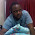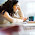## Thursday, 13 October 2011

### Modelling with R: part 4

In part 3, we ran a logistic model to determine the probability of default of a customer. We also saw the outputs and tried to judge the performance of the model b plotting the ROC curve. Let's try a different approach today. How about a decision tree? For starters, we being a simple decision tree with no priors.

##########-------------2.2: Recursive partitioning------------###############

## 2.2.1: Decision tree with no priors  ##

What is recursive? How is partitioning done? How does partitioning translate to predictions? What are priors? Let's try and decode these big words that we have written above.

Partitioning means splitting the data into subsets based on attributes. Partitioning typically happens in a top-down approach by choosing a variable, or an attribute, that is the "best" attribute to split the data. This "best" variable would be one that splits the data into homogeneous subsets with each subset having the same category of the target variable, i.e, either all zeros or all ones. It is in the way of choosing this "best" variable that different tree construction algorithms differ. For example, CART, uses Gini coefficient to choose the best variable. I read that ID3 and C4.5 use the concept of entropy, but I really don't know how these function.

After a subset is formed, the splitting process continues in a "recursive" manner and the recursion halts when the subset at a node has the same value for the target variable.

To run a decision tree we need to call the "rpart" library.
library(rpart)

We use a recursive partioning tree model to predict the likelihood of default.
t1.noprior <- rpart(default ~
amt.fac +
age.fac +
duration +
chk_acct +
history +
purpose +
sav_acct +
employment +
install_rate +
pstatus +
other_debtor +
time_resid +
property +
other_install +
housing +
other_credits +
job +
num_depend +
telephone +
foreign
, data = dev)
plot(t1.noprior)
# Plots the trees
text(t1.noprior)
# Adds the labels to the trees.

Before we score the validation data, we need to prune the tree to ensure that we haven't over fitted the data.
In order to prune the data we need to find the appropriate complexity parameter. The ideal value of the complexity parameter in this case would then be the one that minimizes the X-error.

We can find this value as
printcp(t1.noprior)
# Here we see that the value is 0.013514.

We can write a small script to find this value
t1.noprior.cp <- t1.noprior\$cptable[which.min(t1.noprior\$cptable[, "xerror"]), "CP"]
t1.noprior.prune <- prune(t1.noprior,t1.noprior.cp)

We need to score the pruned tree model the same way we did for the Logistic model.
val\$t1.yhat <- predict(t1.noprior.prune, val, type = "prob")

Now notice the difference between the two predictions- one of the Logistic model and the other of the tree model. The logistic model predictions have only column, which gives the probability that the person will default, or mathematically, the probability of y being equal to 1. On the other hand the tree model predictions give two columns, first for y being equal to zero and second column for y being equal to 1. Hence, for plotting the ROC curve we need to take only the probability of y being equal to 1.

We will also plot the ROC curve for this tree.
t1.scores <- prediction(val\$t1.yhat[,2], val\$default)
t1.perf <- performance(t1.scores, "tpr", "fpr")

# Plot the ROC curve
plot(t1.perf, col = "green", lwd = 1.5)

Now how do we compare the performance of this decision tree model with our earlier logistic model. One simple way would be to plot the two ROCs in the same graph and visually guess it.

# Add the ROC curve of the logistic model and the diagonal line
plot(m1.perf, col = "red", lwd = 1, add = TRUE)
abline(0, 1, lty = 8, col = "grey")
legend("bottomright", legend = c("tree", "logit"), col = c("green", "red"), lwd = c(1.5,1))

Similarly we can calculate the KS statistic as well
ks1.tree <- max(attr(t1.perf, "y.values")[] - (attr(t1.perf, "x.values")[]))
ks1.tree

Another way to compare the performance of two models, especially when the dependent variable is binary, is the Area Under the Curve or the "AUC"

# AUC for the logistic model
m1.auc <- performance(m1.scores, "auc")
m1.auc

# AUC for the decision tree
t1.auc <- performance(t1.scores, "auc")
t1.auc

1.I've had better success with ctree() in the party package than with rpart()

2.ctree() is next on the list Andrew. Just thought of detailing most, if not all, possible options.

Thanks. :)

3.I get this when I run the 'predict' funtion: Invalid prediction for rpart object

The predictions seem to come out fine, but the error message worries me. Great post, very informative! Thanks for taking the time to do it!

4.Thanks Christopher.

I really don't know why/how you are getting the error. What seems odd is that you are getting the error as well the outcome of the function predict().

Typically, if you get an error, the function will not have an outcome. I can maybe help if you share your script.

5.Hi
I am new to R and i was wondering if you could provide guidance to score new sets of data on a daily basis.

In SAS logistic regression we save the model and use it to score the new dataset.

I am trying to use R for a decision tree model but did not know how to do the scoring daily

6.I get Invalid prediction message at this stage for this part

val\$t1.yhat <- predict(t1.noprior.prune, val, type = "prob")

7.This comment has been removed by the author.

8.This comment has been removed by the author.

9.thanks for this.

At first I tried to use that tutorial by Sharma and didn't manage at all. I found a lot of data sources with slightly different data, and so i tried to look for tutorials and found many. Some of them, for example, using outdated libraries (maybe in comparison to the current R version), etc. Thanks for giving a complete "time-proof" tutorial. I hope i will be able to go back and read the other tutorials on this subject too.

10.well explained .Keep updating Artificial intelligence Online Trining